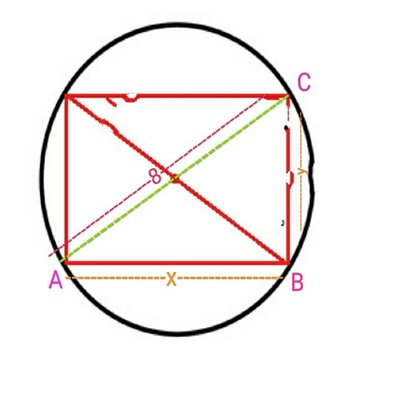# A rectangle is inscribed in a circle with a radius 4 inches. If the length of the rectangle is...

## Question:

A rectangle is inscribed in a circle with a radius 4 inches. If the length of the rectangle is decreasing at the rate of 3 inches per second, how fast is the area changing at the instant when the length is 4 inches?

## Area of Rectangle:

Area of rectangle is defined as the product of the length and width of rectangle.

{eq}A = x\times y\\ {/eq}

We will find the rate of change of area by differentiating the area(A) with respect to time(t).

To find the rate of change of area

Given

The length of the rectangle is decreasing at the rate {eq}\frac{dx}{dt} = 3 \ inch/sec {/eq}

Length of rectangle (x) = 4 inch

Let x be the length and y be the width of rectangle.

Area of rectangle is

{eq}A = x\times y\\ {/eq}Apply phythargeon theroem in {eq}\triangle ABC {/eq} using

{eq}x^2 + y^2 = (8)^2 \\ y ^2 = 64 - x^2 \\ y = \sqrt{64 - x^2} \\ {/eq}

Substituting value of y in area A

{eq}A = x\times \sqrt{64 - x^2} \\ \text{Differentiate with respect to t} \\ \frac{dA}{dt} = (\frac{x(-2x)}{2\sqrt{64-x^2}} + \sqrt{64 - x^2})\frac{dx}{dt} \\ \frac{dA}{dt} = (\frac{-x^2}{\sqrt{64-x^2}} + \sqrt{64 - x^2})\frac{dx}{dt} \\ \text{Substituting value of x and } \ \frac{dx}{dt} \\ \frac{dA}{dt} = (\frac{-(4)^2}{\sqrt{64-(4)^2}} + \sqrt{64 - (4)^2})3 \\ \frac{dA}{dt} = (\frac{-16}{\sqrt{48}} + \sqrt{48})3 \\ \frac{dA}{dt} = (\frac{32}{\sqrt{48}})3 \\ \frac{dA}{dt} = \frac{96}{\sqrt{48}} \\ \frac{dA}{dt} = \frac{24}{\sqrt{3}} \\ \frac{dA}{dt} = 8\sqrt{3} \ inch^2/sec \\ {/eq}